+

# One-Step Linear Equations

##### Rating:
(0)
Author: Sophia Tutorial
##### Description:

Solve one-step linear algebraic questions.

(more)
Tutorial

what's covered
In this lesson, we will solve single-step algebraic equations. By practicing these equations, you will be strengthening your problem solving skills. Specifically, this lesson will cover:
1. Variables and Properties of Equality
2. Inverse Operations
3. Isolating the Variable

## 1. Variables and Properties of Equality

Before we begin to solve algebraic equations, we should review what a variable is. A variable, represented with a letter in an equation, is an unknown value that you are trying to find. This unknown value is represented by a letter; it can be any letter, but the most commonly used is x. It could be represented by anything—a box, a big question mark, etc.—so don’t let the letter scare you.

Before we can solve for the unknown value, we need to discuss Properties of Equality. There are several properties of equality that help to solve equations or determine the variable in an equation:

• The addition property states that if a equals b, and c is any number, then a plus c is equal to b plus c. Adding c on both sides of the equation still provides a true statement.
formula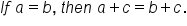• The subtraction property states that if a equals b, and c is any number, then a minus c is equal to b minus c. Subtracting c from both sides of the equation still provides a true statement.
formula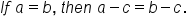• The multiplication property states that if a is equal to b, and c is any number, then a times c is equal to b times c. Multiplying by c on both sides of the equation still provides a true statement.
formula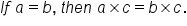• The division property states that if a is equal to b, and c is any non-zero number (you can’t divide a number by 0), then a divided by c is equal to b divided by c. Dividing by c on both sides of the equation still provides a true statement.
formula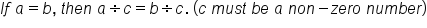big idea
In general, these properties state that whatever is done on one side of the equal sign must be done on the other side to maintain an equation, or a true statement.

terms to know

Variable
An unknown value that we are trying to find.
Rule of Equality
Any operation performed on one side of the equation must be performed on the other side, in order to keep quantities equal in value.

## 2. Inverse Operations

Now that we understand properties of equality, we are one step closer to solving for a variable. To do this, we use inverse operations. Inverse operations are pairs of operations that undo each other, or cancel each other out. Addition and subtraction are a pair of inverse operations, because they undo each other, or cancel each other out.

EXAMPLE

As you can see in the following example, if you start with 3 and add 5, this equals 8. However, if you start with 8 and subtract 5, you are back to your original value of 3. Therefore, adding 5 and subtracting 5 undo each other. Similarly, you can see this is true if you look at 12 minus 8, which equals 4. However, if you start with 4 and add 8, you are back to your original value of 12.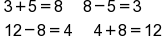Multiplication and division are also inverse operations, because they cancel each other out.

EXAMPLE

We know that 2 multiplied by 7 equals 14, but 14 divided by 7 will bring you back to your original value of 2. Therefore, multiplying by 7 and dividing by 7 undo each other. Similarly, 18 divided by 6 equals 3, but if you start with 3 and multiply by 6, you are back to your original value of 18.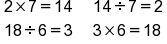## 3. Isolating the Variable

Finding the solution or solving most equations involves isolating a variable, or in other words, getting the unknown value by itself. To do this, you want to rearrange your equation so that the variable is by itself on one side of the equation, and everything else is on the other side. To rearrange your equation, you can use the operations that are the inverse of the operations appearing in the equation.

Suppose you want to solve the following equation. To do this, you’ll need to isolate the variable x on the left side of the equation.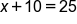Because you are adding 10 to the variable x, you need to use the inverse operation to addition, which is subtraction, to cancel out the plus 10. Therefore, you’re going to subtract 10 on the left side of the equation, which means you also need to subtract 10 on the right side of the equation. On the left side of the equation, the +10 - 10 equals 0. So, the x is isolated by itself of the left side of the equation.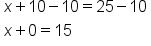hint
Remember, whatever is done on one side of the equal sign must be done on the other side in order to maintain an equation, or a true statement.

Now you have the variable x on the left and 15 on the right, so your solution is x equals 15.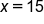EXAMPLE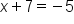The 7 is added to the x.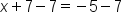Subtract 7 from both sides to cancel the 7 and isolate the x on the left side of the equation.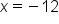Our Solution

try it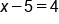The 5 is negative, or subtracted from x.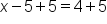Add 5 to both sides.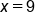Our Solution

Multiplication and division problems use the same method of isolation using the inverse operation. For example, if the equation has a value multiplied by the variable, you would use the opposite function, division, to isolate the variable. Remember, if there is no operation between the number and the variable, we assume the operation is multiplication.

EXAMPLE

With a multiplication problem, we get rid of the number by dividing on both sides. For example consider the following: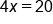Variable is multiplied by 4.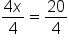Divide both sides by 4 to isolate the x on the left side of the equation.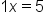4 divided by 4 equals 1.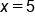Our Solution
As you can see, we arrive at our solution of x = 5.
With multiplication problems it is very important that care is taken with signs. If x is multiplied by a negative, then we will divide by a negative. This is shown in the next example:

EXAMPLE

In division problems, we get rid of the denominator by multiplying on both sides. Consider our next example.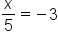Variable is divided by 5.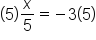Multiply both sides by 5.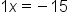On the left side of the equation, we have 5 divided by 5 which equals 1. The x is isolated.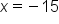Our Solution

try it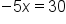Variable is multiplied by -5.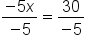Divide both sides by -5.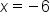Our Solution

try it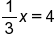Our Equation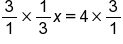Because the x is multiplied by 1/3, we need to use the inverse operation of division to cancel it out. This may look complicated so another way to cancel it out is to multiply it by the reciprocal, 3/1.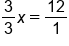Multiplying by 3/1 on the left side of the equation equals 3/3 times x. Multiplying by 3/1 on the right side equals 12/1.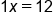Simplify the equation.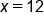Our Solution

### IN CONTEXT

Suppose Jamie scored 18 goals during 9 games of soccer. He wants to know how many goals he scored, on average, per game. You can write an equation to represent the situation.

You can multiply the 9 games of soccer by x, your variable, which represents how many goals he scored per game. Lastly, you know that this will equal the total number of goals he scored, 18.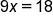Because the x is multiplied by 9, you need to divide by 9 on both sides of the equation to isolate the x. This simplifies to be x on the left side of the equation, and 2 on the right side of the equation. Therefore, your solution is x equals 2, which means that Jamie scored, on average, 2 goals per game.### IN CONTEXT

Suppose we were told that Rachel earned \$40,000 a year for some number of years and ended up making a total of \$280,000 over that time period. For how many years did Rachel work? Can you determine an equation to represent the problem and solve the equation?

Here, we know how much Rachel earns each year, but we are told that this was for "some number of years," which alerts us that the number of years worked would be a variable. We can also determine that if we multiply the number of years worked with her salary each year, we can set that number equal to the total amount earned. Using this equation, we can then solve the problem.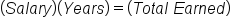Our Equation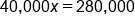x = years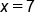Divide both sides by 40,000.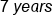Our Solution

### IN CONTEXT

Paula is going to visit her grandmother who lives 455 miles away. Assuming Paula never stops and averages 70 mph, how long will it take to get to her grandmother’s?

To solve this problem, you can use the following formula:

formula
Distance = Rate X Time
If you know two of the values, you can always solve for the unknown third value. Let’s plug in what we know and solve. The distance is 455 miles. The rate is 70 mph. Because we know the distance and rate, our variable for this equation will be: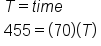Now solve for T.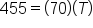Isolate the variable by doing the inverse operation.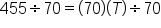Because the variable is currently multiplied by 70, the opposite would be to divide the variable by 70.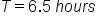Our Solution

This is one of many real-life examples of how we use variables to solve problems in everyday life.

summary
Today you learned that a variable, which is represented with a letter in an equation, is an unknown value that you are trying to find or solve for. You also learned about several properties of equality, which can be used to help solve for a variable in an equation. Lastly, you learned that to solve for a variable, you want to isolate the variable on one side of the equation and move everything else to the other side, and you can do this using inverse operations. Practicing these equations strengthens your ability to solve a variety of problems.

Best of luck in your learning!

Terms to Know
Rule of Equality

Any operation performed on one side of the equation must be performed on the other side, in order to keep quantities equal in value.

Variable

An unknown value that we are trying to find.

Rating Header